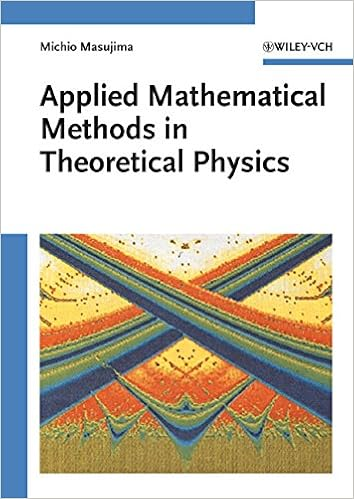## Monday, October 12, 2015

### Applied Mathematical Methods in Theoretical Physics 1st Edition by Michio MasujimaAll there is to know about functional analysis, integral equations and calculus of variations in a single volume.
This advanced textbook is divided into two parts: The first on integral equations and the second on the calculus of variations. It begins with a short introduction to functional analysis, including a short review of complex analysis, before continuing a systematic discussion of different types of equations, such as Volterra integral equations, singular integral equations of Cauchy type, integral equations of the Fredholm type, with a special emphasis on Wiener-Hopf integral equations and Wiener-Hopf sum equations.
After a few remarks on the historical development, the second part starts with an introduction to the calculus of variations and the relationship between integral equations and applications of the calculus of variations. It further covers applications of the calculus of variations developed in the second half of the 20th century in the fields of quantum mechanics, quantum statistical mechanics and quantum field theory.
Throughout the book, the author presents over 150 problems and exercises - many from such branches of physics as quantum mechanics, quantum statistical mechanics, and quantum field theory - together with outlines of the solutions in each case. Detailed solutions are given, supplementing the materials discussed in the main text, allowing problems to be solved making direct use of the method illustrated. The original references are given for difficult problems. The result is complete coverage of the mathematical tools and techniques used by physicists and applied mathematicians
Intended for senior undergraduates and first-year graduates in science and engineering, this is equally useful as a reference and self-study guide.